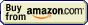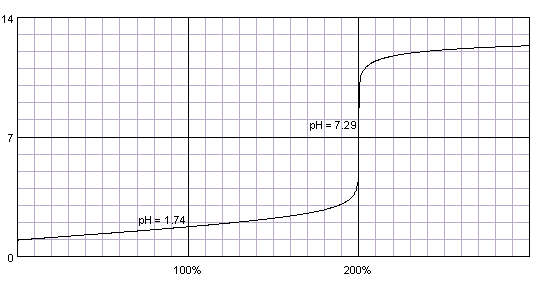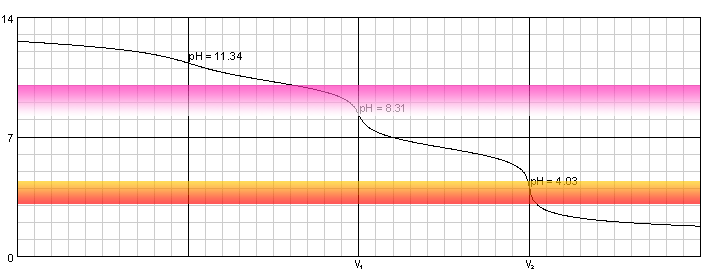# Acid-base titration## Acid-base titration » Titration of polyprotic substances and mixtures

BATE pH calculator

operating systems:
XP, Vista, 7, 8, 10

€24.95 - approximately \$33

By clicking Buy Now! you will continue to the FastSpring checkout page where payment will be taken, and your order fulfilled by FastSpring, our trusted reseller, and Merchant of Record.

30-day money back guarantee!

Titration of polyprotic acids (or bases) requires more attention than titration of monoprotic ones. There are two reasons for that.

First, polyprotic acid can have more then one inflection point on the titration curve. As in laboratory practice possible pH change during titration is limited to about 7-8 units at most, that means steep part of the titration curve - when split between two end points - must be short. As we have seen in the end point detection section it doesn't help to achieve good accuracy of titration.

Second, quite often without precise analysis it is not obvious what is stoichiometry of the reaction. For example, when we titrate phosphoric acid with strong base, we will be never able to observe third end point (titration curve is completely flat in this area). Thus we have to select indicator that will allow titration of either only first proton, or two protons - and calculate reaction stoichiometry aaccordingly. Even then determination won't be easy - see titration curve below.

Titration curves of polyprotic acids, presented below, show these problems.0.1M solution of sulfuric acid titrated with 0.1M solution of strong base. pKa1=-3, pKa2=2. Titration curve calculated with BATE - pH calculator.

Sulfuric acid - while its second proton is much less acidic than the first one - is strong enough so that both protons get titrated together. There is only one, nice and high steep part of the titration curve. Everything that was told about indicator selection for titration of strong acids still holds.0.1M solution of maleic acid titrated with 0.1M solution of strong base. pKa1=1.83, pKa2=6.07. Titration curve calculated with BATE - pH calculator.

Carefully selecting both pKa values we can calculate better titration curve, with two almost identical end points. However, this is curve of a real substance - maleic acid. Curve at the first inflection point is not steep enough to be used for end point determination.0.1M solution of citric acid titrated with 0.1M solution of strong base. pKa1=3.13, pKa2=4.76, pKa3=6.40. Titration curve calculated with BATE - pH calculator.

Citric acid has three relatively similar dissociation constants, thus instead of giving three (or at least two) separate end points, it has a long ramp, at which buffering effect of the first and second dissociation steps doesn't allow for fast rise of pH.0.1M solution of phosphoric acid titrated with 0.1M solution of strong base. pKa1=2.15, pKa2=7.20, pKa3=12.35. Titration curve calculated with BATE - pH calculator.

In the case of phosphoric acid first two protons are similar in strength to protons in maleic acid, thus initially titration curve looks similar, with two separate end points. Third dissociation constant is so small, that even after adding large excess of 0.1M titrant over 20% of the acid is in the form of HPO42-.

Note, that in almost all titration curves presented above, pH changes close to the end point are relatively slow compared to the titration curves of monoprotic acids (even weak, monoprotic acid). That means it is very difficult to precisely determine the end point of the titration.

While in many cases more then one end point makes titration difficult, it allows simultaneous determination of NaOH and Na2CO3 in one solution in so called Warder titration.Warder titration - solution of 0.1M NaOH and 0.1M Na2CO3 titrated with 0.1M solution of strong acid. Carbonic acid dissociation constants: pKa1=6.37, pKa2=10.25.

Solution of sodium hydroxide and disodium carbonate contains three bases - OH-, CO32- and HCO3-. First two are much stronger, so they are neutralized first. First equivalence point is at pH 8.31, almost exactly at pH where phenolphthalein starts (or - in this case - ceases) to be visible. Second end point is at pH 4.03, right in the middle of methyl orange color change pH range.

At the first equivalence point we have a solution of NaHCO3, its pH can be calculated - using formula derived in the lecture on pH of amphiprotic salt - as average of pKa1 and pKa2. pH of the second end point is calculated simply as result of the dissociation of carbonic acid.

During titration of the mixture we first add phenolphthalein to the solution and titrate it till pink color disappears, noting the first end point volume (let's call it V1). Then we add methyl orange and titrate solution to the intermediate color of the indicator. Let's call the second end point volume V2.

V1 is the volume of titrant that was necessary for completion of two reactions:

NaOH + HCl → NaCl + H2O

Na2CO3 + HCl → NaCl + NaHCO3

V2-V1 is the hydrogen carbonate protonation:

NaHCO3 + HCl → NaCl + H2O + CO2

Obviously V2-V1 can be directly used to calculate amount of the carbonate present in the original solution. V1 reflects a sum of NaOH and Na2CO3. As we know V2-V1 is the volume needed for Na2CO3 protonation, we can easily calculate that 2V1-V2 is the volume of the titrant necessary to neutralize just NaOH.

Note, that Warder titration requires high accuracy of the end point detection, as curve near the equivalence point is not too steep and pH changes are relatively slow.